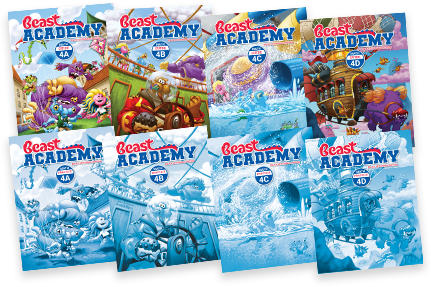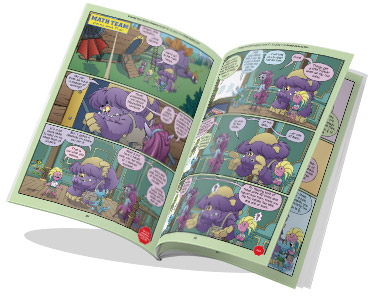By Jason Batterson and Shannon Rogers
Illustrated by Erich Owen

# Level 4 Math Books

Ages 10‑12By Jason Batterson and Shannon Rogers
Illustrated by Erich Owen\$118.00

## Level 4 Books Information

Designed in an engaging comic-book style format, our Level 4 curriculum includes the Guide books and Practice books for all four units (4A, 4B, 4C, 4D).

• The Guide book is a full-color paperback textbook, which teaches concepts in a comic-book style.
• The Practice book complements the Guide book and includes 300+ problems ranging from introductory-level exercises to challenging puzzles and word problems.Level 4 has four units: A, B, C and D. Topics build upon each other from unit to unit.

4A
4B
4C
4D
Shapes
Definitions, angle measurement, parallel and perpendicular lines, symmetry.
Counting
Counting lists, independent events, Venn diagrams, factorials, counting pairs.
Factors
Prime and composite numbers, divisibility, prime factorization, factor trees.
Fractions
Multiplying fractions and mixed numbers. Division by unit fractions.
Multiplication
The distributive property, multi-digit multiplication, using algorithms, the units digit.
Division
Special quotients, dividing multiples of powers of 10, long division, divisibility.
Fractions
Adding, subtracting, and converting fractions and mixed numbers.
Decimals
Place value, converting between fractions and decimals, comparing and ordering decimals.
Exponents
Computing powers, order of operations, perfect squares, binary numbers (base-2).
Logic
Deductive reasoning, logic puzzles, grid puzzles, liars and truth-tellers.
Integers
Understanding negatives. Adding and subtracting positive and negative integers.
Probability
Counting strategies review, coins, dice, random events, and geometric probability.

We recommend taking the Level 4A Placement Test to determine if your student is ready to take Beast Academy Level 4.

Solutions to the placement test are found at the end of the test. Your student may also take the Level 4B, 4C, or 4D placement tests to determine readiness per unit.Get the guide books for sure, because they are endearing and interesting and adorable and wonderful and they make your kid fall in love with doing math (even if they already love math).

Kjersti, BA parent